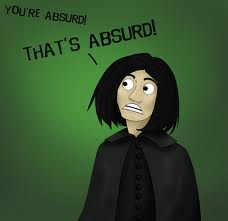# Absurd PrimeIf a prime $p$ can be expressed as the difference of square of two positive integers, then find the remainder, when $p^{2}+138$ is divided by $4$.

×

Problem Loading...

Note Loading...

Set Loading...